强化学习q学习求最值by Thomas Simonini 通过托马斯·西蒙尼(Thomas Simonini)
通过Q学习更深入地学习强化学习 (Diving deeper into Reinforcement Learning with Q-Learning)
What Q-Learning is 什么是Q学习 How to implement it with Numpy 如何使用Numpy实施  大图：骑士与公主 (The big picture: the Knight and the Princess)
Let’s say you’re a knight and you need to save the princess trapped in the castle shown on the map above. 假设您是一名骑士，您需要保存困在上面地图所示城堡中的公主。
You can move one tile at a time. The enemy can’t, but land on the same tile as the enemy, and you will die. Your goal is to go the castle by the fastest route possible. This can be evaluated using a “points scoring” system. 您一次只能移动一个图块。 敌人不能，但是和敌人降落在同一块地上，你会死。   您的目标是尽可能快地走城堡。 可以使用“积分”系统进行评估。
You lose -1 at each step (losing points at each step helps our agent to be fast). 您   每步损失-1(每步损失点   帮助我们的代理更快)。 If you touch an enemy, you lose -100 points, and the episode ends. 如果碰到敌人，您将失去-100分，情节结束。 If you are in the castle you win, you get +100 points. 如果您在城堡中获胜，您将获得+100分。 The question is: how do you create an agent that will be able to do that? 问题是：如何创建能够做到这一点的代理？
Here’s a first strategy. Let say our agent tries to go to each tile, and then colors each tile. Green for “safe,” and red if not. 这是第一个策略。 假设我们的经纪人尝试转到每个图块，然后为每个图块着色。 绿色表示“安全”，否则表示红色。
Then, we can tell our agent to take only green tiles. 然后，我们可以告诉我们的代理商只拿绿砖。
But the problem is that it’s not really helpful. We don’t know the best tile to take when green tiles are adjacent each other. So our agent can fall into an infinite loop by trying to find the castle! 但是问题在于它并没有真正的帮助。 我们不知道绿色瓷砖彼此相邻时采取的最佳瓷砖。   因此我们的经纪人可以通过尝试找到城堡陷入无限循环！
Q表介绍 (Introducing the Q-table)
Here’s a second strategy: create a table where we’ll calculate the maximum expected future reward, for each action at each state. 这是第二种策略：创建一个表，在该表中，我们将为每个州的每个动作计算最大的预期未来奖励。
Thanks to that, we’ll know what’s the best action to take for each state. 因此，我们将知道对每个州采取的最佳措施是什么。
Each state (tile) allows four possible actions. These are moving left, right, up, or down. 每个状态(平铺)都允许四个可能的操作。 它们在向左，向右，向上或向下移动。
In terms of computation, we can transform this grid into a table. 在计算方面，我们可以将此网格转换为表格。
This is called a Q-table (“Q” for “quality” of the action). The columns will be the four actions (left, right, up, down). The rows will be the states. The value of each cell will be the maximum expected future reward for that given state and action. 这称为Q表 (“ Q”代表动作的“质量”)。 列将是四个动作(左，右，上，下)。 这些行将是状态。 每个单元格的值将是给定状态和动作的最大预期未来回报。
Each Q-table score will be the maximum expected future reward that I’ll get if I take that action at that state with the best policy given. 如果我在给出最佳政策的情况下在该州采取该行动，则每个Q表得分将是我将获得的最大预期未来奖励。
Why do we say “with the policy given?” It’s because we don’t implement a policy. Instead, we just improve our Q-table to always choose the best action. 我们为什么说“给出了政策？” 这是因为我们没有执行政策。 相反，我们只是改进我们的Q表，以始终选择最佳操作。
Think of this Q-table as a game “cheat sheet.” Thanks to that, we know for each state (each line in the Q-table) what’s the best action to take, by finding the highest score in that line. 将此Q表视为游戏“备忘单”。 多亏了这一点，我们知道了每个状态(Q表中的每一行)所采取的最佳措施，即找出该行中的最高得分。
Yeah! We solved the castle problem! But wait… How do we calculate the values for each element of the Q table? 是的 我们解决了城堡问题！ 但是等等……我们如何计算Q表中每个元素的值？
To learn each value of this Q-table, we’ll use the Q learning algorithm. 要学习此Q表的每个值， 我们将使用Q学习算法。
Q学习算法：学习动作值函数 (Q-learning algorithm: learning the Action Value Function)
The Action Value Function (or “Q-function”) takes two inputs: “state” and “action.” It returns the expected future reward of that action at that state. 动作值函数(或“ Q函数”)接受两个输入：“状态”和“动作”。 它返回该状态下该动作的预期将来奖励。
We can see this Q function as a reader that scrolls through the Q-table to find the line associated with our state, and the column associated with our action. It returns the Q value from the matching cell. This is the “expected future reward.” 我们可以看到这个Q函数，它是一个读取器，它滚动Q表以查找与我们的状态关联的行以及与我们的动作关联的列。 它从匹配的单元格返回Q值。 这是“预期的未来奖励”。
But before we explore the environment, the Q-table gives the same arbitrary fixed value (most of the time 0). As we explore the environment, the Q-table will give us a better and better approximation by iteratively updating Q(s,a) using the Bellman Equation (see below!). 但是在探索环境之前，Q表会给出相同的任意固定值(大多数情况下为0)。 当我们探索环境时，通过使用Bellman方程迭代更新Q(s，a) ， Q表将为我们提供越来越好的近似值(请参见下文！)。
Q学习算法过程 (The Q-learning algorithm Process)
Step 1: Initialize Q-valuesWe build a Q-table, with m cols (m= number of actions), and n rows (n = number of states). We initialize the values at 0. 第1步：初始化Q值我们构建一个Q表，其中包含m个 cols(m =动作数)和n行(n =状态数)。 我们将值初始化为0。
Step 2: For life (or until learning is stopped)Steps 3 to 5 will be repeated until we reached a maximum number of episodes (specified by the user) or until we manually stop the training. 步骤2：终生(或直到学习停止) ，将重复步骤3至5，直到达到最大发作次数(由用户指定)或直到我们手动停止训练为止。
Step 3: Choose an actionChoose an action a in the current state s based on the current Q-value estimates. 步骤3：选择一个动作根据当前的Q值估算值，选择一个处于当前状态s的动作。
But…what action can we take in the beginning, if every Q-value equals zero? 但是……如果每个Q值等于零，我们在一开始可以采取什么措施？
That’s where the exploration/exploitation trade-off that we spoke about in the last article will be important. 那就是我们在上一篇文章中谈到的勘探/开采权衡的重要性所在。
The idea is that in the beginning, we’ll use the epsilon greedy strategy: 想法是，一开始，   我们将使用epsilon贪婪策略：
We specify an exploration rate “epsilon,” which we set to 1 in the beginning. This is the rate of steps that we’ll do randomly. In the beginning, this rate must be at its highest value, because we don’t know anything about the values in Q-table. This means we need to do a lot of exploration, by randomly choosing our actions. 我们指定一个探索率“ε”，在开始时将其设置为1。 这是我们将随机执行的步骤的速度。 首先，该速率必须为最高值，因为我们对Q表中的值一无所知。 这意味着我们需要通过随机选择行动来进行大量探索。 We generate a random number. If this number > epsilon, then we will do “exploitation” (this means we use what we already know to select the best action at each step). Else, we’ll do exploration. 我们生成一个随机数。 如果该数字> epsil o n，那么我们将进行“剥削”(这意味着我们将使用我们已经知道的方法在每个步骤中选择最佳操作)。 否则，我们将进行探索。 The idea is that we must have a big epsilon at the beginning of the training of the Q-function. Then, reduce it progressively as the agent becomes more confident at estimating Q-values. 想法是，在训练Q功能时，我们必须有一个很大的epsilon。 然后，随着代理对估计Q值变得更有信心时，逐渐减小它。 Steps 4–5: Evaluate!Take the action a and observe the outcome state s’ and reward r. Now update the function Q(s,a). 步骤4–5：评估！ 采取行动a并观察结果状态s'并奖励r。 现在更新函数Q(s，a)。
We take the action a that we chose in step 3, and then performing this action returns us a new state s’ and a reward r (as we saw in the Reinforcement Learning process in the first article). 我们采取在步骤3中选择的动作a ，然后执行此动作将为我们返回新状态s'和奖励r (如我们在第一篇文章的强化学习过程中所看到的)。
Then, to update Q(s,a) we use the Bellman equation: 然后，要更新Q(s，a)，我们使用Bellman方程：
The idea here is to update our Q(state, action) like this: 这里的想法是像这样更新我们的Q(state，action)：
New Q value =    Current Q value +    lr * [Reward + discount_rate * (highest Q value between possible actions from the new state s’ ) — Current Q value ]Let’s take an example: 让我们举个例子：
One cheese = +1 一种奶酪= +1 Two cheese = +2 两块奶酪= +2 Big pile of cheese = +10 (end of the episode) 大堆奶酪= +10(情节结束) If you eat rat poison =-10 (end of the episode) 如果您吃了鼠药= -10(发作结束) Step 1: We init our Q-table 步骤1：我们建立Q表
Step 2: Choose an action From the starting position, you can choose between going right or down. Because we have a big epsilon rate (since we don’t know anything about the environment yet), we choose randomly. For example… move right. 第2步：选择一个动作从起始位置，您可以选择向右还是向下。 因为我们的epsilon率很高(因为我们对环境一无所知)，所以我们随机选择。 例如……向右移动。
We found a piece of cheese (+1), and we can now update the Q-value of being at start and going right. We do this by using the Bellman equation. 我们找到了一块奶酪(+1)，现在我们可以更新起点和终点的Q值。 我们通过使用Bellman方程来做到这一点。
Steps 4–5: Update the Q-function 步骤4–5：更新Q功能
First, we calculate the change in Q value ΔQ(start, right) 首先，我们计算Q值的变化量ΔQ(开始，右) Then we add the initial Q value to the ΔQ(start, right) multiplied by a learning rate. 然后，我们将初始Q值与ΔQ(start，right)相乘乘以学习率。 Think of the learning rate as a way of how quickly a network abandons the former value for the new. If the learning rate is 1, the new estimate will be the new Q-value. 可以将学习率视为网络放弃新旧价值的一种方式。 如果学习率是1，则新的估计值将是新的Q值。
Good! We’ve just updated our first Q value. Now we need to do that again and again until the learning is stopped. 好！ 我们刚刚更新了第一个Q值。 现在，我们需要一次又一次地这样做，直到学习停止。
实施Q学习算法 (Implement a Q-learning algorithm)
We made a video where we implement a Q-learning agent that learns to play Taxi-v2 with Numpy. 我们制作了一个视频，其中我们实现了一个Q学习代理，该代理学习了如何与Numpy玩Taxi-v2。
Now that we know how it works, we’ll implement the Q-learning algorithm step by step. Each part of the code is explained directly in the Jupyter notebook below. 现在我们知道了它的工作原理，我们将逐步实现Q学习算法。 下面的Jupyter笔记本中直接解释了代码的每个部分。
You can access it in the Deep Reinforcement Learning Course repo. 您可以在“ 深度强化学习课程”存储库中访问它。
回顾... (A recap…)
Q-learning is a value-based Reinforcement Learning algorithm that is used to find the optimal action-selection policy using a q function. Q学习是一种基于值的强化学习算法，用于使用aq函数查找最佳的动作选择策略。 It evaluates which action to take based on an action-value function that determines the value of being in a certain state and taking a certain action at that state. 它基于操作值函数来评估要采取的操作，该函数确定处于某个状态并在该状态下执行某个操作的值。 Goal: maximize the value function Q (expected future reward given a state and action). 目标：最大化价值函数Q(给定状态和动作的预期未来回报)。 Q table helps us to find the best action for each state. Q表可帮助我们找到每个状态的最佳操作。 To maximize the expected reward by selecting the best of all possible actions. 通过选择所有可能的动作中的最佳动作来最大化预期的回报。 The Q come from quality of a certain action in a certain state. Q来自品质   在特定状态下的特定动作。 Function Q(state, action) → returns expected future reward of that action at that state. 函数Q(状态，动作)→返回该动作在该状态的预期未来回报。 This function can be estimated using Q-learning, which iteratively updates Q(s,a) using the Bellman Equation 可以使用Q学习估计该函数，Q学习使用Bellman方程迭代地更新Q(s，a)。 Before we explore the environment: Q table gives the same arbitrary fixed value → but as we explore the environment → Q gives us a better and better approximation. 在探索环境之前：Q表给出相同的任意固定值→但在我们探索环境时→Q给出了越来越好的近似值。 That’s all! Don’t forget to implement each part of the code by yourself — it’s really important to try to modify the code I gave you. 就这样！ 不要忘了自己实现代码的每个部分-尝试修改我给您的代码非常重要。
Try to add epochs, change the learning rate, and use a harder environment (such as Frozen-lake with 8x8 tiles). Have fun! 尝试添加纪元，更改学习率，并使用更艰苦的环境(例如带有8x8磁贴的冰冻湖)。 玩得开心！
Next time we’ll work on Deep Q-learning, one of the biggest breakthroughs in Deep Reinforcement Learning in 2015. And we’ll train an agent that that plays Doom and kills enemies! 下次，我们将进行深度Q学习，这是2015年深度强化学习中最大的突破之一。我们还将训练一个扮演末日并杀死敌人的特工！
If you liked my article, please click the ? below as many time as you liked the article so other people will see this here on Medium. And don’t forget to follow me! 如果您喜欢我的文章， 请单击“？”。 您可以根据自己喜欢该文章的次数在下面进行搜索，以便其他人可以在Medium上看到此内容。 并且不要忘记跟随我！
If you have any thoughts, comments, questions, feel free to comment below or send me an email: hello@simoninithomas.com, or tweet me @ThomasSimonini. 如果您有任何想法，意见，问题，请在下面发表评论，或给我发送电子邮件：hello@simoninithomas.com或向我发送@ThomasSimonini信息 。
Keep learning, stay awesome! 继续学习，保持卓越！
使用Tensorflow进行深度强化学习课程？ (Deep Reinforcement Learning Course with Tensorflow ?️)
? Syllabus ？ 教学大纲
? Video version ？ 视频版本
Part 1: An introduction to Reinforcement Learning 第1部分： 强化学习简介
Part 2: Diving deeper into Reinforcement Learning with Q-Learning 第2部分： 通过Q-Learning更深入地学习强化学习
Part 3: An introduction to Deep Q-Learning: let’s play Doom 第3部分： 深度Q学习简介：让我们玩《毁灭战士》
Part 3+: Improvements in Deep Q Learning: Dueling Double DQN, Prioritized Experience Replay, and fixed Q-targets 第3部分+： 深度Q学习中的改进：双重DQN，优先体验重播和固定Q目标
Part 4: An introduction to Policy Gradients with Doom and Cartpole 第4部分： Doom和Cartpole的策略梯度简介
Part 5: An intro to Advantage Actor Critic methods: let’s play Sonic the Hedgehog! 第5部分： 优势演员评论家方法简介：让我们玩刺猬索尼克吧！
Part 6: Proximal Policy Optimization (PPO) with Sonic the Hedgehog 2 and 3 第6部分： 使用刺猬索尼克2和3的近距离策略优化(PPO)
Part 7: Curiosity-Driven Learning made easy Part I
第七部分： 好奇心驱动学习变得简单
翻译自: https://www.freecodecamp.org/news/diving-deeper-into-reinforcement-learning-with-q-learning-c18d0db58efe/强化学习q学习求最值
展开全文python java 人工智能 算法
• Q学习，很有帮助.jie shao le Q-learning de ji ben shiyong
• Q学习（Q-learning） 强化学习中有个很重要的递归关系，贝尔曼方程（Bellman Equation）： Qπ(st,at)=E[r+γE[Qπ(st+1,at+1)]]Q^\pi(s_t,a_t)=E[r+\gamma E[Q^\pi(s_{t+1},a_{t+1})]]Qπ(st​,at​)=E[r+γE[Qπ...
最近想做个汇总，所以这里简单写一下。
Q学习（Q-learning）
强化学习中有个很重要的递归关系，贝尔曼方程（Bellman Equation）：
$Q^\pi(s_t,a_t)=E[r+\gamma E[Q^\pi(s_{t+1},a_{t+1})]]$
这个公式实际上也揭露了状态的马尔科夫性质，也就是下一个状态只与当前状态有关。强化学习中大多数方法都是基于这个公式，Q学习也一样。Q学习的更新公式如下：
$Q(s,a)=Q(s,a)+\alpha[r+\gamma max_{a'}Q(s,a)-Q(s,a)]$
可以看见不同于贝尔曼方程中使用下一个状态的期望来估计当前Q值，Q学习中使用的是下一个状态的最大Q值来估计当前状态Q值。这是由于强化学习的agent目标是最大化累积奖赏，也就是Q值。但是这会带来的问题是 高估 。这篇文章是没有涉及解决这个问题的，Sutton的书中提到的解决方法是Double Q-learning，结合深度学习就是DDQN，我们组老师也在17年IJCAI发了一篇解决这个问题的文章Weighted Double Q-learning。下面是Q-learning和Double Q-learning的算法：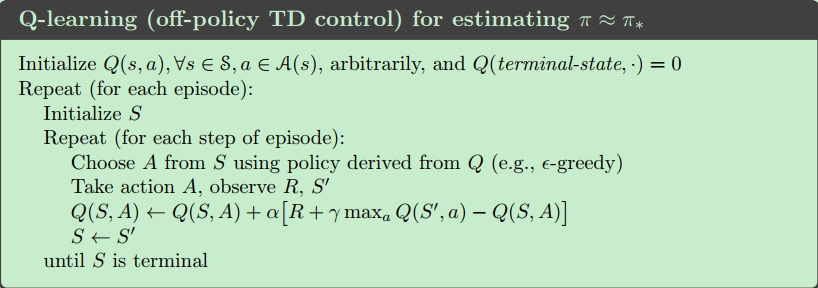在double Q-learning中会定义两个Q函数，选择动作时是在两者之和的基础上进行$\epsilon-greedy$选择，在更新时这两个函数是交互使用的，若一个Q1更新则Q2则作为max评估，这样在一定程度上可以解决 高估 问题。(简单理解就是因为Q2下的max并不是Q1下的max)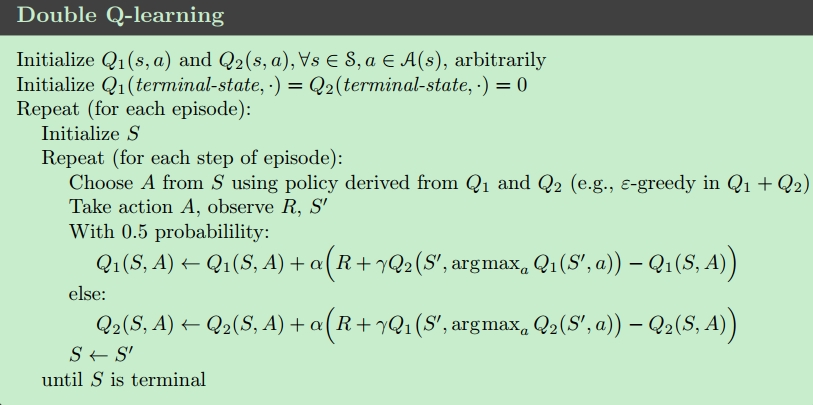深度Q学习（DQN）
DQN中使用神经网络来作为Q值的逼近函数。权重和偏置用 $\theta$ 表示。损失函数表示为：$L(s,a|\theta_i)\approx (r+\gamma max_{a'}Q(s',a|\theta_i)-Q(s,a|\theta_i))^2$
梯度更新公式：
$\theta_{i+1}=\theta_i+\alpha\ \bigtriangledown_\theta L(\theta_i)$
但是若使用相同的网络来生成下一个目标Q值和估计当前Q值，会导致振荡性甚至发散。还有就是，深度学习要求样本之间相互独立且同分布，但强化学习样本并不满足这个条件。因此DQN中使用三个技巧来来解决这一系列问题：经验回放，目标网络和自适应性学习率调整方法。（事实上当使用函数逼近时（比如神经网络作为逼近函数），强化学习会表现的不稳定甚至发散，主要原因是：序列观察值之间具有关性，对于Q网络的微小更新会导致策略明显的变化，以及action value Q(s,a)和target action value $r+\gamma max Q(s',a')$之间的关联性。这三点都是一定会存在的问题，不可比避免的。DQN中使用的经验回放主要是一定程度上打破序列之间的相关性，而目标网络是解决Q值和目标Q值之间的相关性的（原文Human-level control through deep reinforcement
learning第四段）。但是对于第二个原因，并由和好的解决，所以可以朝着这个方向拓展。）
经验回放，是指在Agent与环境交互过程中，经验会以$(s_t,a_t,r_t,s_{t+1})$的形式存放在经验池D中，每次训练会从D中随机抽样出一批数据来进行训练，这样就可以在一定程度上消除样本之间的相关性。目标网络是指，DQN中使用两个网络，一个网络是当前网络，与环境交互，并不断更新。另一个网络是目标网络，它并不与环境交互，也不在每个时间步进行更新，而是每隔一定时间步才会更新，每次更新都是把当前网络参数直接赋值给它。
具体的操作是每此训练时，比如第$i$次迭代，一个小批量经验（minibatch）经验$e_t=(s_t,a_t,r_t,s_{t+1})$会从经验池中随机抽样出来。损失函数定义为:$L_i(\theta_i)=E_{(s,a,r,s')\sim D}[(y_i-Q(s,a|\theta_i))^2]$其中$y_i=r+\gamma max_{a'}Q^-(s',a'|\theta^-)$这里$Q^-$就是目标网络。每过一定时间步就会将$\theta$赋值给$\theta^-$。伪代码如下：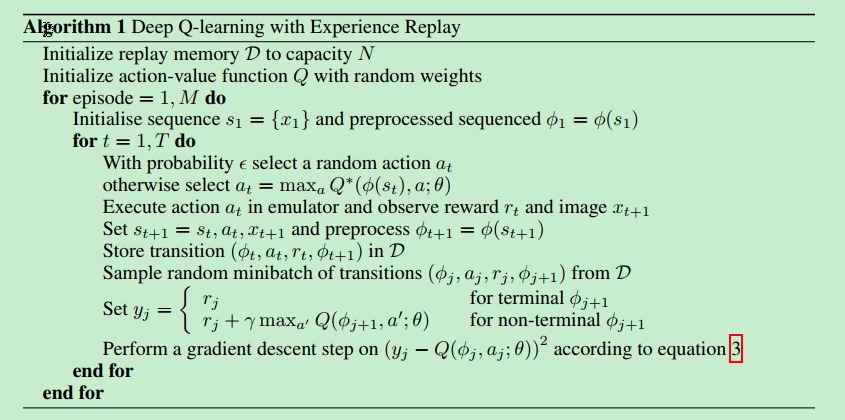展开全文DQN
• 从各种网站在下载到的Q学习算法集成包，有matlab示例，有C示例，有Java示例，有C++示例。总有一个算法是你需要模拟和借鉴的，需要研究强化学习或增强学习算法的人不容错过。。。真心话，要不是我需要下载别的资源...
• Q-learning基本原理，即通过几个例子初步了解q学习
• 实现的内容很简单，存为.m文件可以直接在matlab上运行，就是利用Q学习（Q learning）完成自主路径寻优简单示例，并进行可视化，Q学习部分参考了如上链接中的内容，供大家交流学习使用，请多提宝贵意见 如图为最终...
参考链接：https://blog.csdn.net/Maggie_zhangxin/article/details/73481417

实现的内容很简单，存为.m文件可以直接在matlab上运行，就是利用Q学习（Q learning）完成自主路径寻优简单示例，并进行可视化，Q学习部分参考了如上链接中的内容，供大家交流学习使用，请多提宝贵意见

如图为最终路径，红色方框代表机器人，绿色区域代表障碍，中间底部位置（图示红色方框位置）为目标位置，蓝色为运动轨迹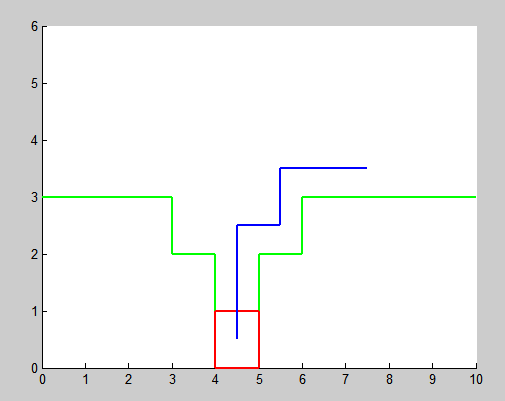本程序对训练过程进行了可视化，方便理解学习过程，代码如下：

clc;
clear all;
% define state
R=ones(60,60)*-inf;
for i=1:30
if i-10>0
R(i,i-10)=0;
end
if i+10<31
R(i,i+10)=0;
end
if mod(i,10)~=1
R(i,i-1)=0;
end
if mod(i,10)~=0;
R(i,i+1)=0;
end
end
R(24,34)=0;R(25,35)=0;R(26,36)=0;
R(34,24)=0;R(35,25)=0;R(36,26)=0;
R(34,35)=0;R(35,36)=0;R(35,34)=0;R(36,35)=0;
R(35,45)=0;R(45,35)=0;
R(45,55)=100;R(55,45)=0;

% reinforcement learning parameters
gamma=0.9;
q=zeros(size(R));     % q matrix
q1=ones(size(R))*inf; % previous q matrix
count=0;

% visualize obstacle
axis([0,10,0,6]);
hold on;
plot([0,3],[3,3],'g','linewidth',2);
plot([6,10],[3,3],'g','linewidth',2);
plot([3,3],[2,3],'g','linewidth',2);
plot([6,6],[2,3],'g','linewidth',2);
plot([4,4],[0,2],'g','linewidth',2);
plot([5,5],[0,2],'g','linewidth',2);
plot([3,4],[2,2],'g','linewidth',2);
plot([5,6],[2,2],'g','linewidth',2);

% intial state
y=randperm(30);
state=y(1);

% q learning
tic
for episode=0:50000

qma=max(q(state,:));
if qma~=0
x=find(q(state,:)==qma);
else
x=find(R(state,:)>=0);
end
% choose action
if size(x,1)>0
x1=RandomPermutation(x);
x1=x1(1);
end
% update q matrix
qMax=max(q,[],2);
q(state,x1)=R(state,x1)+gamma*qMax(x1);

Y(i)=5.5-floor((x1-1)/10);
X(i)=0.5+rem(x1-1,10);
% visualization
A=plot([X(i)-0.5,X(i)+0.5],[Y(i)-0.5,Y(i)-0.5],'r-','linewidth',2);
B=plot([X(i)-0.5,X(i)+0.5],[Y(i)+0.5,Y(i)+0.5],'r-','linewidth',2);
C=plot([X(i)-0.5,X(i)-0.5],[Y(i)-0.5,Y(i)+0.5],'r-','linewidth',2);
D=plot([X(i)+0.5,X(i)+0.5],[Y(i)-0.5,Y(i)+0.5],'r-','linewidth',2);
pause(0.05);

% break if converged: small deviation on q for 1000 consecutive
if sum(sum(abs(q1-q)))<0.0001 && sum(sum(q))>190
if count>500,
episode        % report last episode
break          % for
else
count=count+1; % set counter if deviation of q is small
end
else
q1=q;
count=0;
end

if(R(state,x1)==100)
y=randperm(30);
state=y(1);
pause(0.4);
else
state=x1;
end
delete(A);
delete(B);
delete(C);
delete(D);

end
toc
%normalization
g=max(max(q));
if g>0,
q=100*q/g;
end


需要配合函数使用，函数 RandomPermutation.m 在参考链接中给出，原作者：

(http://people.revoledu.com/kardi/)

这里一并给出

function y=RandomPermutation(A)
%%%%%%%%%%%%%%%%%%%%%%%%%%%%%%%%%
% return random permutation of matrix A
% unlike randperm(n) that give permutation of integer 1:n only,
% RandomPermutation rearrange member of matrix A randomly
% This function is useful for MonteCarlo Simulation,
%  Bootstrap sampling, game, etc.
%
% (http://people.revoledu.com/kardi/)
%
% example: A = [ 2, 1, 5, 3]
% RandomPermutation(A) may produce [ 1, 5, 3, 2] or [ 5, 3, 2, 3]
%
% example:
% A=magic(3)
% RandomPermutation(A)
%%%%%%%%%%%%%%%%%%%%%%%%%%%%%%%%%%%
[r,c]=size(A);
b=reshape(A,r*c,1);       % convert to column vector
x=randperm(r*c);          % make integer permutation of similar array as key
w=[b,x'];                 % combine matrix and key
d=sortrows(w,2);          % sort according to key
y=reshape(d(:,1),r,c);    % return back the matrix


--------------------- 2019-4-24 更新 ----------------------------------

感谢scxDAWN的提醒，示意图里面画训练结束后最终轨迹的程序附在下面，蓝线表示走过的轨迹，如果迭代次数不够多，可能会出现不太合理的轨迹，如果只希望看最终结果而不关心过程，可以把之前 q-learning 训练程序中画图的部分都去掉，可以很快得到最终收敛的结果，然后用下面程序检验训练效果即可：


axis([0,10,0,6]);
%grid on;
%axis equal;
hold on;
plot([0,3],[3,3],'g','linewidth',2);
plot([6,10],[3,3],'g','linewidth',2);
plot([3,3],[2,3],'g','linewidth',2);
plot([6,6],[2,3],'g','linewidth',2);
plot([4,4],[0,2],'g','linewidth',2);
plot([5,5],[0,2],'g','linewidth',2);
plot([3,4],[2,2],'g','linewidth',2);
plot([5,6],[2,2],'g','linewidth',2);

st=randperm(30);
s=st(1);
%s=28;

i=1;
while s~=55

%商
Y(i)=5.5-floor((s-1)/10);
%余数
X(i)=0.5+rem(s-1,10);
%plot(X,Y,'*');

A=plot([X(i)-0.5,X(i)+0.5],[Y(i)-0.5,Y(i)-0.5],'r-','linewidth',2);
B=plot([X(i)-0.5,X(i)+0.5],[Y(i)+0.5,Y(i)+0.5],'r-','linewidth',2);
C=plot([X(i)-0.5,X(i)-0.5],[Y(i)-0.5,Y(i)+0.5],'r-','linewidth',2);
D=plot([X(i)+0.5,X(i)+0.5],[Y(i)-0.5,Y(i)+0.5],'r-','linewidth',2);
pause(0.2);
if i>1;
plot([X(i-1),X(i)],[Y(i-1),Y(i)],'b-','linewidth',2);
end

qm=max(q(s,:));
if qm~=0
ac=find(q(s,:)==qm);
else
ac=find(R(s,:)>=0);
end

if size(ac,2)>1
act=RandomPermutation(ac);
act=act(1);
else
act=ac;
end

delete(A);
delete(B);
delete(C);
delete(D);

s=act;
i=i+1;
end

%商
Y(i)=5.5-floor((s-1)/10);
%余数
X(i)=0.5+rem(s-1,10);
A=plot([X(i)-0.5,X(i)+0.5],[Y(i)-0.5,Y(i)-0.5],'r-','linewidth',2);
B=plot([X(i)-0.5,X(i)+0.5],[Y(i)+0.5,Y(i)+0.5],'r-','linewidth',2);
C=plot([X(i)-0.5,X(i)-0.5],[Y(i)-0.5,Y(i)+0.5],'r-','linewidth',2);
D=plot([X(i)+0.5,X(i)+0.5],[Y(i)-0.5,Y(i)+0.5],'r-','linewidth',2);

if i>1;
plot([X(i-1),X(i)],[Y(i-1),Y(i)],'b-','linewidth',2);
end


展开全文• 讲述Q学习算法基本原理，并通过几个小例子初步了解q学习算法应用。
• Q学习的matlab代码。自己写的并且配了详细注释，很好理解。
• 强化学习 Q学习算法matlab实现 多智能体模拟仿真 算法基本思路概括，引导。强化学习的q学习算法,能够通过此算法，使得某种动作不断得到加强，希望对那些想使用Q学习算法的人有所帮助
• Please follow this link to understand the basics of Reinforcement Learning.... Let’s explain various components before Q... 让我们在Q学习之前解释各种组件。 基于策略的基于价值的RL (Policy-based vs value...
Please follow this link to understand the basics of Reinforcement Learning.请点击此链接以了解强化学习的基础知识。
Let’s explain various components before Q-learning. 让我们在Q学习之前解释各种组件。
基于策略的基于价值的RL
(Policy-based vs value-based RL)
In policy-based RL, the random policy is selected initially and find the value function of that policy in the evaluation step. Then find the new policy from the value function computed in the improve step. The process repeats until it finds the optimal policy. In this type of RL, the policy is updated directly 在基于策略的RL中，首先选择随机策略，然后在评估步骤中找到该策略的价值函数。 然后从改进步骤中计算出的价值函数中找到新策略。 重复该过程，直到找到最佳策略为止。 在这类RL中，政策会直接更新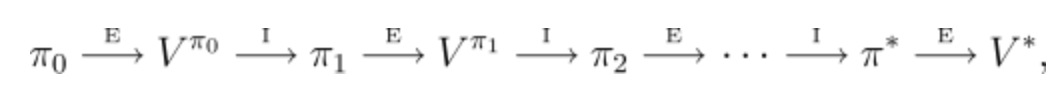In a value-based approach, the random value function is selected initially, then find new value function. This process repeated until it finds the optimal value function. The intuition here is the policy that follows the optimal value function will be optimal policy. Here, the policy is implicitly updated through value function. In Q-learning updating the value function(Q-value) to find the optimal policy在基于值的方法中，当前作随机值函数被选择最初，然后寻找新的值的功能。 重复此过程，直到找到最佳值函数为止。 直觉是遵循最优值功能的策略将是最优策略。 在这里，该策略通过值函数隐式更新。 在Q学习中更新值函数(Q值)以找到最佳策略
RL算法的三种基本方法(Three basic approaches of RL algorithms)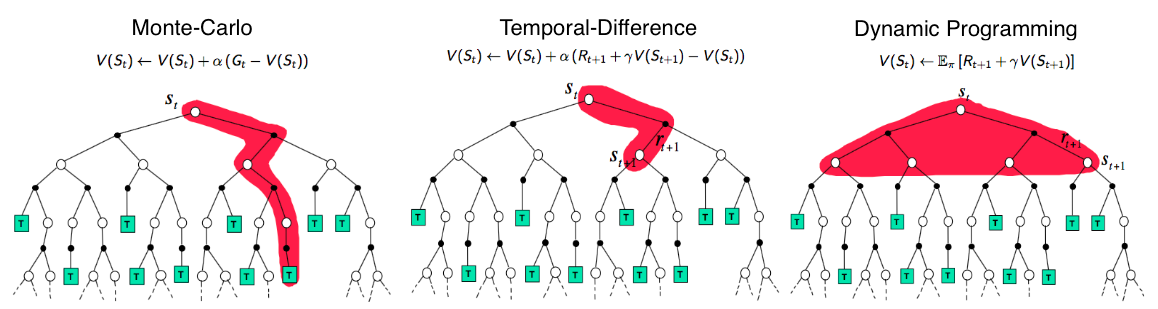These algorithms are basis for the various RL algorithms to solve MDP. SourceTemporal-Difference(TD) learning is a combination of Monte-Carlo and Dynamic Programming (DP) methods. Like the Monte-Carlo method, TD method can learn directly from raw experience without a model of the environment’s dynamics. Like DP, TD methods update estimates based in part on other learned estimates, without waiting for a final outcome (they call bootstrapping). Basically, in Q-learning, we are using 1 step TD learning approach. It means, we update the Q value by taking a single action, rather than waiting till the end of the episode to update the value function. This will be more clear when we introduce the equation later in the article. 时差(TD)学习是蒙特卡罗方法和动态编程(DP)方法的结合。 像蒙特卡洛方法一样，TD方法可以直接从原始经验中学习，而无需建立环境动力学模型。 像DP一样，TD方法也部分基于其他学习的估计来更新估计，而无需等待最终结果(它们称为自举)。 基本上，在Q学习中，我们使用1步TD学习方法。 这意味着，我们通过执行单个操作来更新Q值，而不是等到情节结束才更新值函数。 当我们在本文后面介绍方程式时，这一点将更加清楚。
价值功能
(Value functions)
The value function measures the goodness of the state(state-value) or how good is to perform an action from the given state(action-value).值函数可衡量状态(状态值)的优劣或根据给定状态(动作值) 执行某项操作的质量。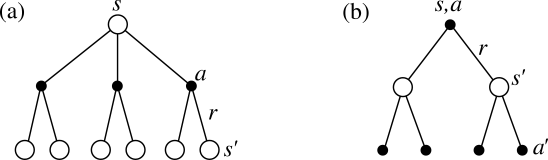Backup diagram of stat-value and action-value functions: a) state-value b) action-value. Source: 状态值函数
(state-value function)
The state-value Vπ(s) is the expected total reward, starting from state s and acts according to policy π.状态值Vπ(s)是从状态s开始的预期总报酬，并根据策略π进行操作。
If the agent uses a given policy π to select actions, the corresponding value function is given by: 如果代理使用给定策略π选择操作，则相应的值函数由下式给出：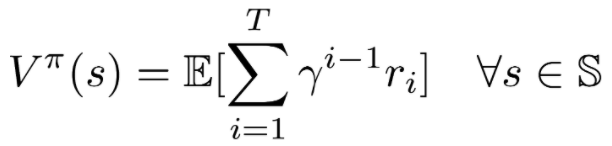Optimal state-value function: It has high possible value function compared to other value function for all states 最佳状态值函数：与所有状态的其他值函数相比，它具有较高的可能值函数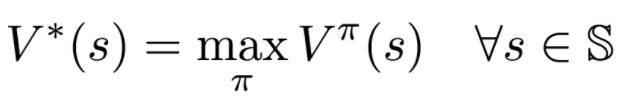In a value-based RL, If we know optimal value function, then the policy that corresponds to optimal value function is optimal policy 𝛑*.在基于价值的RL中，如果我们知道最优价值函数，那么对应于最优价值函数的策略就是最优策略𝛑 *。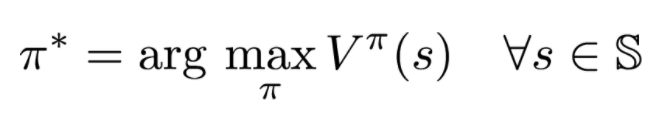动作值功能
(Action-value function)
It is the expected return for an agent starting from state s and taking an action a then forever after act according to policy 𝛑. The state can have multiple actions, thus there will be multiple Q value in a state.根据状态starting，从状态s开始采取行动，然后永远采取行动，是代理商的预期收益。 状态可以有多个动作，因此一个状态中会有多个Q值。
The optimal Q-function Q*(s, a) means highest possible Q value for an agent starting from state s and choosing action a. There, Q*(s, a) is an indication for how good it is for an agent to pick action while being in state s. 最佳Q函数Q *(s，a)表示从状态s开始选择动作a的代理的最大可能Q值。 Q *(s，a)表示代理处于状态s时采取行动有多好。
Since V*(s) is the maximum expected total reward when starting from state s, it will be the maximum of Q*(s, a) over possible Q* value of other actions in the state s. Therefore, the relationship between Q*(s, a) and V*(s) is easily obtained as: 由于V *(s)是从状态s开始时的最大预期总奖励，因此它将是状态s中其他动作的可能Q *值的最大值Q *(s，a)。 因此，Q *(s，a)和V *(s)之间的关系很容易获得：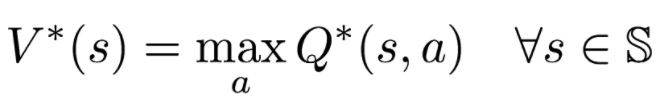and If we know the optimal Q-function Q*(s, a), the optimal policy can be easily extracted by choosing the action a that gives maximum Q*(s, a) for state s. 如果我们知道最优Q函数Q *(s，a)，则可以通过选择为状态s给出最大Q *(s，a)的动作a轻松提取最优策略。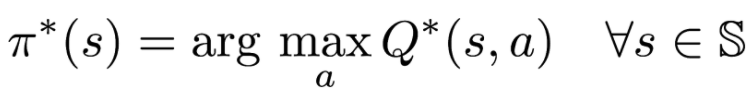Q学习
(Q-learning)
Q learning is a value-based off-policy temporal difference(TD) reinforcement learning. Off-policy means an agent follows a behaviour policy for choosing the action to reach the next state s_t+1 from state s_t. From s_t+1, it uses a policy π that is different from behaviour policy. In Q-learning, we take absolute greedy action as policy π from the next state s_t+1.Q学习是一种基于价值的偏离策略的时间差异(TD)强化学习。 非政策手段 代理遵循一种行为策略，用于选择要从状态s_t到达下一个状态s_t + 1的动作。 从s_t + 1开始，它使用与行为策略不同的策略π。 在Q学习中，我们从下一个状态s_t + 1采取绝对贪婪行为作为策略π。Computation of Q-value in Q-learning. SourceAs we discussed in the action-value function, the above equation indicates how we compute the Q-value for an action a starting from state s in Q learning. It is the sum of immediate reward using a behaviour policy(ϵ-soft, ϵ-greedy or softmax) and from state s_t+1, it takes the absolute greedy action (choose the action that has maximum Q value over other actions). 正如我们在动作值函数中讨论的那样，上面的等式表明了我们如何从Q学习中的状态s开始计算动作a的Q值。 它是使用行为策略(ϵ-soft，ϵ-greedy或softmax)的立即奖励的总和，并且从状态s_t + 1开始，采取绝对贪婪操作(选择与其他操作相比具有最大Q值的操作)。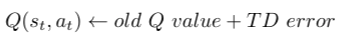Basic update rule in Q-learningIt is important to mention the update rule in Q-learning. New Q value is the sum of old Q value and TD error.重要的是要在Q学习中提及更新规则。 新Q值是旧Q值与TD误差之和。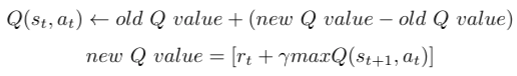Expanding the TD error in Q-learningTD error is computed by subtracting the new Q value from the old Q value.通过从旧Q值中减去新Q值来计算TD误差。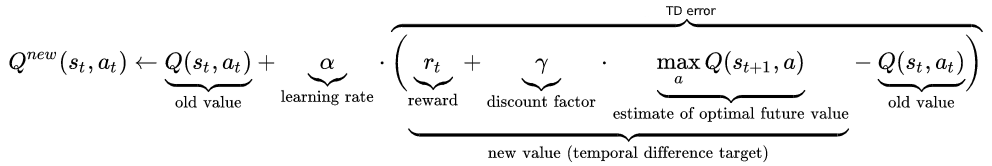Updating rule in Q-learning. SourceThe above equation shows the elaborate view of the updating rule. 上面的等式显示了更新规则的详细视图。
Q表
(Q-table)
We are going to discuss the Q-learning using Q-table. When we use Neural Networks, it is called DQN and we can discuss that in another article.我们将讨论使用Q表的Q学习。 当我们使用神经网络时，它称为DQN，我们可以在另一篇文章中进行讨论。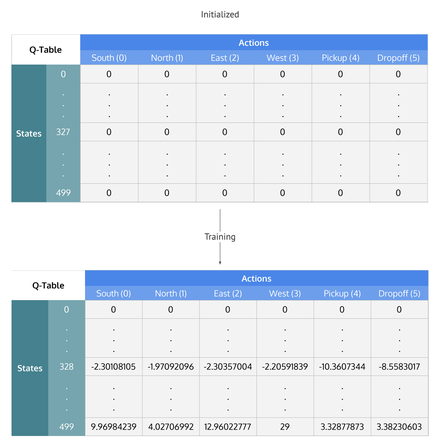Q-table. Source:Q-table contains q values for each and every state-action pair. During the learning process, Q values in the table get updated. Q表包含每个状态动作对的q值。 在学习过程中，表中的Q值会更新。
使用Q表在情节任务中进行Q学习的伪代码
(Pseudo-code for Q-learning in the episodic task using Q-table)
Initialize with Q-table with zero value.用零值的Q表初始化。Episode begins 情节开始
Perform action a_t from state st and observe the next state s_t+1 and reward r从状态st执行动作a_t并观察下一个状态s_t + 1并奖励rCompute the new Q value using the below equation and update the Q-table 使用以下等式计算新的Q值并更新Q表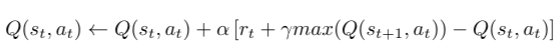Source5. s_t+1 is the new state s_t and repeat steps 3 to 4 until the s_t+1 reaches the terminal state 5. s_t + 1是新状态s_t，重复步骤3到4，直到s_t + 1达到终端状态为止。
6. Episode ends 6.情节结束
7. Repeat steps 2 to 6 until the optimal Q value is reached7.重复步骤2至6，直到达到最佳Q值
In the next article, we can discuss the Deep Q-learning(DQN)在下一篇文章中，我们将讨论深度Q学习(DQN)
If you like my write up, follow me on Github, Linkedin, and/or Medium profile. 如果您喜欢我的文章，请在Github ， Linkedin和/或Medium个人资料上关注我。
翻译自: https://medium.com/intro-to-artificial-intelligence/q-learning-a-value-based-reinforcement-learning-algorithm-272706d835cf
展开全文python 算法 人工智能
• MatLab强化学习代码包，为使用深度Q学习解决网格迷宫问题的代码。 详细说明可参看我的专栏《强化学习与控制》 https://blog.csdn.net/weixin_43723517/category_9676083.html "I thought what I'd do was I'd ...
• 深度Q学习 深度Q学习将深度学习和强化学习相结合，是第一个深度强化学习算法。深度Q学习的核心就是用一个人工神经网络q(s,a;w),s∈S,a∈Aq(s,a;w),s∈\mathcal{S} ,a∈\mathcal{A}q(s,a;w),s∈S,a∈A 来代替动作价值...算法
• 一、从马尔科夫过程到Q学习 # 有一定基础的读者可以直接看第二部分 Q学习（Q-learning）算法是一种与模型无关的强化学习算法，以马尔科夫决策过程（Markov Decision Processes, MDPs）为理论基础。 标准的...Python 强化学习
• 利用MATLAB写的Q学习的程序，同时附件包含Q学习在交通控制中的应用，希望能帮到相关人员
• 用于强化学习，在机器人大赛中用所应用，希望能给大家带来帮组，表述了强化学习方法Q学习在机器人臂章中的应用
• 零、为什么需要深度Q学习 上一期的文章《网格迷宫、Q-learning算法、Sarsa算法》的末尾，我们提到了Q学习固有的缺陷：由于智能体(agent)依赖以状态-动作对为自变量的Q函数表(Q Function Table)来形成对当前状态的...神经网络 人工智能 matlab
• Q学习是常见的强化学习方法，深度Q学习是为了解决传统Q学习的限制（存储空间）而出现的方法，目前多用于对高维数据的学习（深度--可以处理高维数据）。在python中使用tensorflow等框架可轻松实现深度Q学习，但是理解...
• ''' 利用Q学习解决出租车问题 ''' """ 智能体必须在一个位置上接上乘客并在另一个位置放下乘客。 成功放下乘客，那么智能体将会得到奖励+20分，且每经过一 个时间步得到-1分。如果智能体错误搭载和放下，则会得到 -...
• 从强化学习基本概念到Q学习的实现，打造自己的迷宫智能体 2017-04-04 机器之心 选自Medium 作者：Aneek Das 机器之心编译 参与：蒋思源 近年以来，强化学习在人工智能所充当的角色...
• 学习了 Sutton 的《强化学习（第二版）》第6章时序差分学习的控制部分，将笔记提炼如下：Sarsa、Q-learning、期望Sarsa、双 Q 学习强化学习 自动控制 动态规划 人工智能
• ## Q学习的局限性

千次阅读 2017-05-19 21:55:11
1.Q学习是平坦式（ flat）的，不能很好地捕捉任务结构，尤其受维数灾难的约束。 2.利用经典的TD error来one-step更新迭代，达到(near)/optimal ，速度慢！！ 3.对于exploration与exploitation之间的balance没有一...
• 强化学习的经典案例，Q学习法解决迷宫问题的经典Matlab源代码。
• 获取更多资讯，赶快关注上面的...文章目录2.4 强化学习环境 gym 介绍2.4.1 安装2.4.2 FrozenLake-v02.4.2.1 描述2.4.2.2 代码2.5 强化学习算法2.5.1 表格 Q 学习2.5.1.1 代码 2.4 强化学习环境 gym 介绍   这一...
• 入门 | 从Q学习到DDPG，一文简述多种强化学习算法 通常，强化学习的设置由两部分组成，一个是智能体（agent），另一个是环境（environment）。 环境指的是智能体执行动作时所处的场景（例如雅达利游戏中的游戏...
• 以下是强化学习-时间差分学习算法、SARSAmax算法、Q学习算法与神经网络模型相结合的迭代流程图(深度Q学习） 流程的关键点是： 1、流程中动作值函数的逼近方法使用的是神经网络模型（图中含NET的步骤，具体根据需要...机器学习 强化学习 神经网络
• 文章目录第五章 基于时序差分和Q学习的无模型预测与控制5.1 学习目标5.2 TD预测-*- coding: utf-8 -*--*- coding: utf-8 -*- 第五章 基于时序差分和Q学习的无模型预测与控制 在强化学习所有的思想中，时序差分（TD）...强化学习 算法
• Q学习方法抛开了一个显式的策略，直接去学习Q函数，使我们知道在某个特定的状态下执行某一操作效果有多好。但是如果我们使用神经网络来进行拟合可能出现的不收敛现象，这一问题将在所有的使用某些结构（如神经网络）...
• 这是一个完美的Q学习算法C语言程序，外国大神写的，亲测100%零error，零warning无压力！含有大量注释，帮助理解！只需要简单修改几个数字参数，就能输出你想要的Q值表！
• 强化学习的一些相关概念 智能体（Agent）： 智能体对环境进行观察，决策出行动，获得一个从环境返回的奖励 决策（Decision）：意识层面的 行动（Action ， a）：物质层面的 环境（Environment）：与智能体交互的...
• 第5节 编写一个Q学习的小例子 本节使用python编写一个简单的Q学习例子，来体会Q学习中各种参数及更新过程。 最后的效果是什么样的呢？ 这次要编写的是机器人寻宝，是一个线性的环境，o代表机器人，T代表终点，也...python...